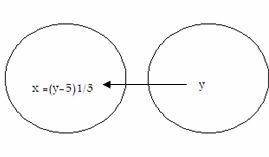Name: ___________________Date:___________________

kwizNET Subscribers, please login to turn off the Ads!
 Email us to get an instant 20% discount on highly effective K-12 Math & English kwizNET Programs!

### High School Mathematics - 24.5 Onto and Into Functions

 The mapping f: A -> B is called an onto function if the set B is entirely used up, i.e if every element of B is the image of atleast one element of A. For every b € B there exists atleast one element a € Asuch that f(a) = bIf function f: A -> B is not onto, that is some of the elements of B remain unmated, then f is called an into function. Remarks: An onto function is also called surjective function or a surjection. A one-one function may be both onto and into. If A and B are finite sets and f: A->B is surjective, then n(B) <= n(A). If n(A) = m, n(B) = m, then the possible number of possible surjective mappings from A to B is m!. : Let A = {-2,-2, 3,3}, B = {4,9} and f: A ->B be a function defined by f(x) = x2, then f is onto because f(-2) = 4, f(-3) = 9, f(3) = 9 i.e f(A) = {4,9} = B. If A and B are finite sets and f: A ->B is surjective, then n(B) <= n(A). If n(A) = m and n(B) - m, then the possible number of possible surjective mappings frm A to B is m!. Example: Let A = {-2, 2, -3, 3}, B = {4,9} and f: A -> B be a function defined by f(x) = x2, then f is onto, because f(-2) and f(2) = 4 and f(-3) and f(3) = 9,i.e f(A) = {4,9} = BA function f: N ->N defined by f(x) = 7x is not an onto function, because f(N) = {7,14,21,..} is not equal to N(co-domain). Method to find surjectivity of s function f: R -> R defined by f(x) = x3 + 5 for all x € R. Choose any arbitrary element y in B. Put f(x) = y Solve the equation f(x) = y for x and obtain x in terms of y. Let x = g(y). If for all values of y € B, the values of x obtained from x = g(y) are in A, then f is onto. If there are some y € B for which x, given by x = g(y), is not in A, then f is not onto. Solution: Let there be an arbitrary element in R. Then f(x) = y = x3 + 5 x = (y -5)1/3. Now for all y € R, (y-5)1/3 is a real number. So for all y € R (co-domain), there exists x = (y - 5)1/3 in R (domain) such that f(x) = x3 + 5Here f: R -> R is an onto function. Directions: Answer the following.Name: ___________________Date:___________________

### High School Mathematics - 24.5 Onto and Into Functions

 Q 1: Given A = {-2, -1, 0, 1, 2} and B = {-3, -1, 1, 5}. list the elements of S = {(x, y): y = 2x2 - 3, x Î A and y Î B}. Is S a function? If so classify it. Answer: Question 2: This question is available to subscribers only!

#### Subscription to kwizNET Learning System costs less than \$1 per month & offers the following benefits:

• Unrestricted access to grade appropriate lessons, quizzes, & printable worksheets
• Instant scoring of online quizzes
• Progress tracking and award certificates to keep your student motivated
• Unlimited practice with auto-generated 'WIZ MATH' quizzes
• Child-friendly website with no advertisements

© 2003-2007 kwizNET Learning System LLC. All rights reserved. This material may not be reproduced, displayed, modified or distributed without the express prior written permission of the copyright holder. For permission, contact info@kwizNET.com
For unlimited printable worksheets & more, go to http://www.kwizNET.com.